SBOS848B December   2017  – October 2019

PRODUCTION DATA.

1. Features
2. Applications
3. Description
1.     Device Images
4. Revision History
5. Pin Configuration and Functions
6. Specifications
7. Detailed Description
1. 7.1 Overview
2. 7.2 Functional Block Diagram
3. 7.3 Feature Description
4. 7.4 Device Functional Modes
8. Applications and Implementation
1. 8.1 Application Information
2. 8.2 Typical Applications
1. 8.2.1 Bidirectional Window Comparator
2. 8.2.2 Solenoid Low-Side Current Sensing
9. Power Supply Recommendations
10. 10Layout
11. 11Device and Documentation Support
12. 12Mechanical, Packaging, and Orderable Information

#### Package Options

Refer to the PDF data sheet for device specific package drawings

• DGS|10
• DSG|8
• DSG|8

### 6.6 Typical Characteristics

at TA = 25°C, VS = 5 V, VIN+ = 12 V, and alert pullup resistor = 10 kΩ (unless otherwise noted)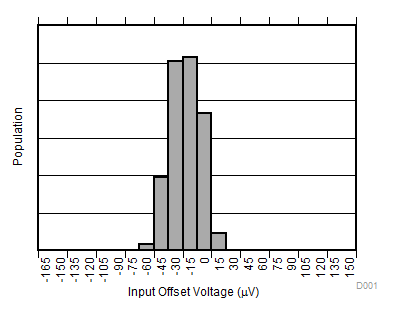VIN+ = 0 V
Figure 1. Input Offset Voltage Production Distribution (INA381A1)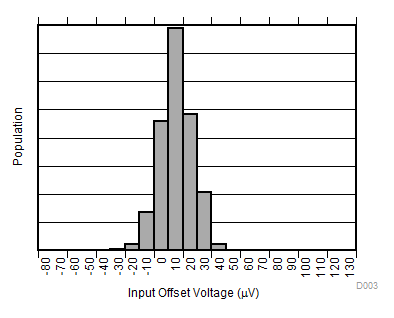VIN+ = 0 V
Figure 3. Input Offset Voltage Production Distribution (INA381A3)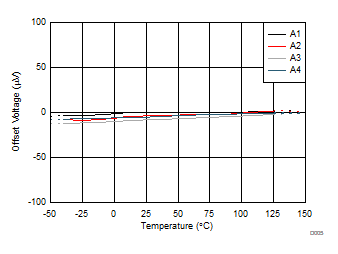VIN+ = 0 V
Figure 5. Offset Voltage vs Temperature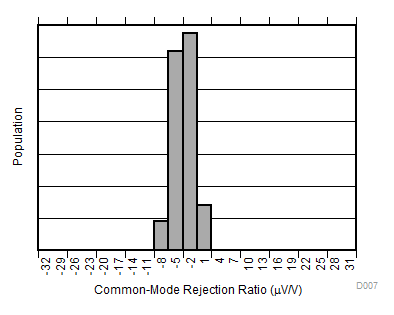Figure 7. Common-Mode Rejection Production Distribution (INA381A2)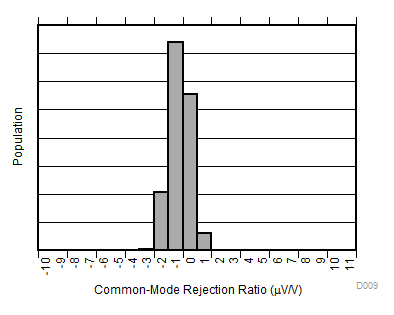Figure 9. Common-Mode Rejection Production Distribution (INA381A4)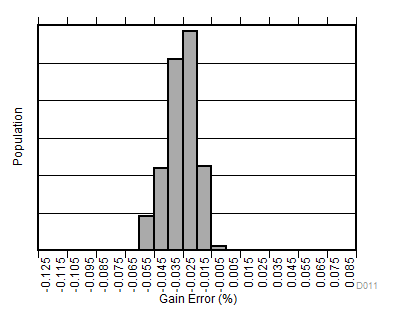Figure 11. Gain Error Production Distribution (INA381A1)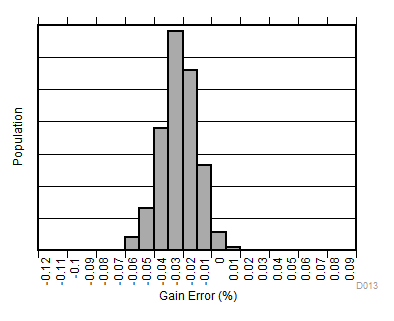Figure 13. Gain Error Production Distribution (INA381A3)Figure 15. Gain Error vs Temperature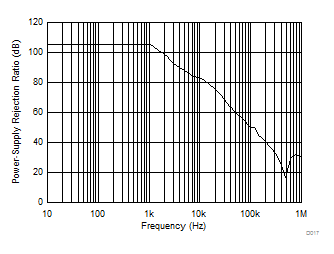Figure 17. Power-Supply Rejection Ratio vs Frequency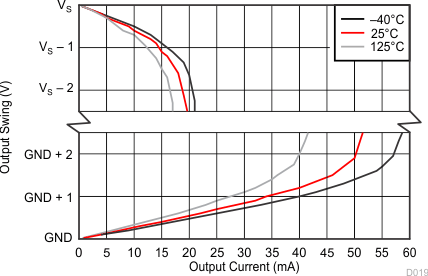Figure 19. Output Voltage Swing vs Output CurrentSupply voltage = 0 V
Figure 21. Input Bias Current vs Common-Mode Voltage (Both Inputs, Shutdown)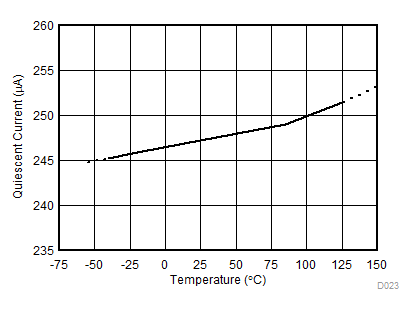Figure 23. Quiescent Current vs Temperature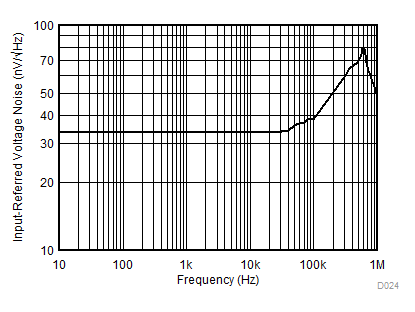Figure 25. Input-Referred Voltage Noise vs Frequency
(INA381A3 Devices)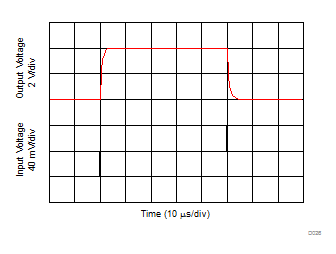80-mVPP input step
Figure 27. Step Response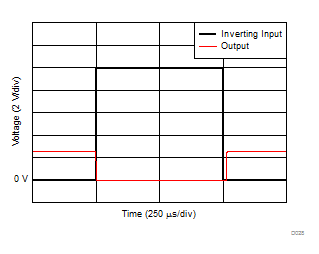Figure 29. Inverting Differential Input OverloadFigure 31. Start-Up Response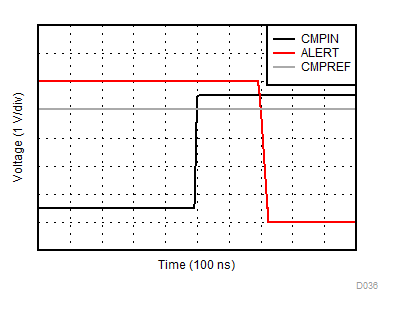Figure 33. Comparator Propagation Delay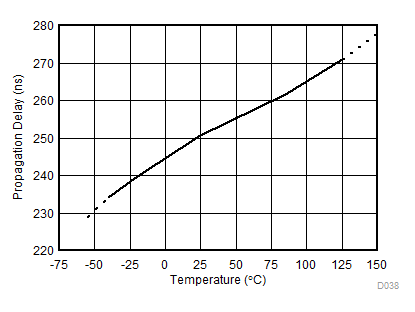Figure 35. Comparator Propagation Delay vs Temperature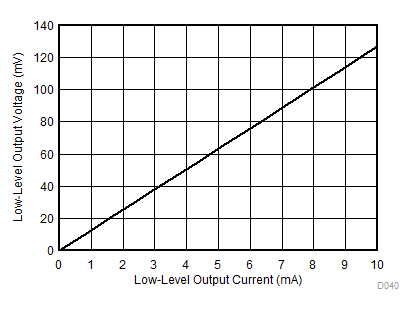Figure 37. Low-Level Output Voltage vs Low-Level Output Current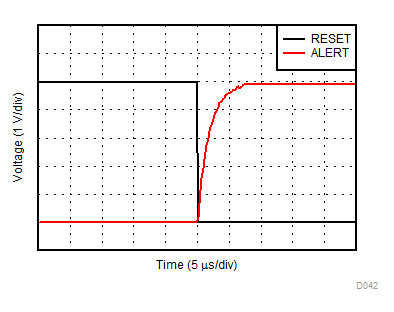Figure 39. Reset and Alert Voltage Response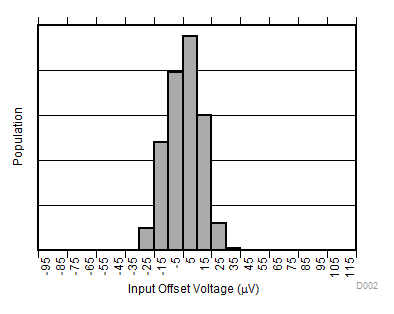VIN+ = 0 V
Figure 2. Input Offset Voltage Production Distribution (INA381A2)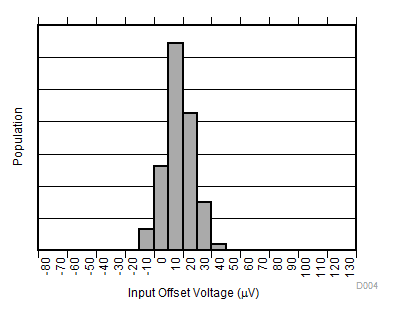VIN+ = 0 V
Figure 4. Input Offset Voltage Production Distribution (INA381A4)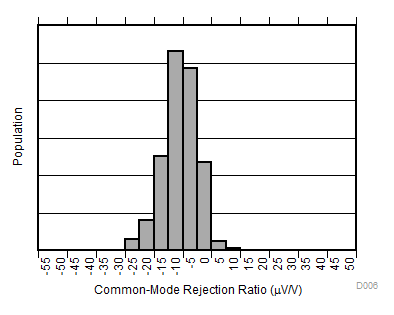Figure 6. Common-Mode Rejection Production Distribution (INA381A1)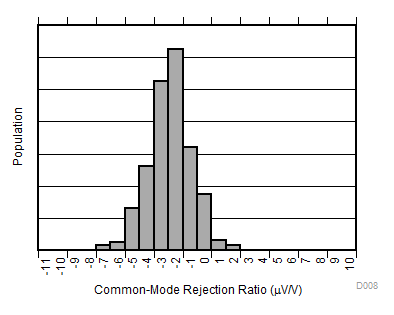Figure 8. Common-Mode Rejection Production Distribution (INA381A3)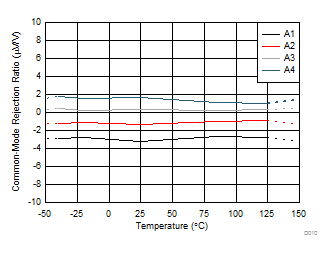Figure 10. Common-Mode Rejection Ratio vs Temperature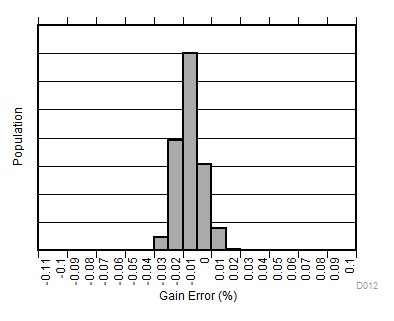Figure 12. Gain Error Production Distribution (INA381A2)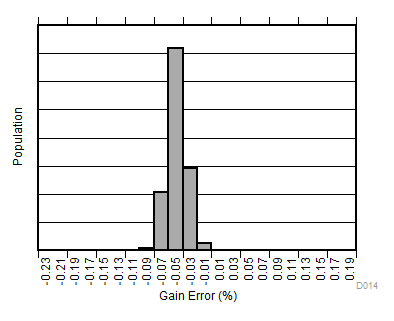Figure 14. Gain Error Production Distribution (INA381A4)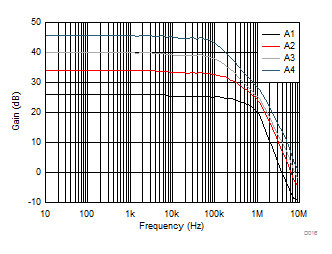Figure 16. Gain vs Frequency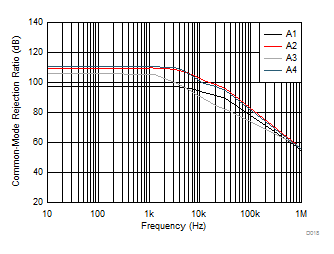Figure 18. Common-Mode Rejection Ratio vs Frequency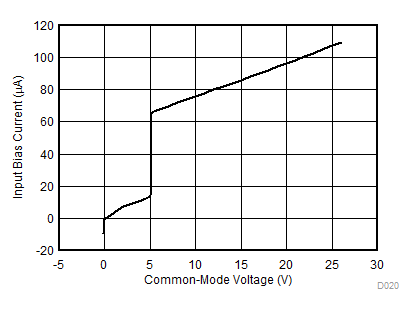Supply voltage = 5 V
Figure 20. Input Bias Current vs Common-Mode Voltage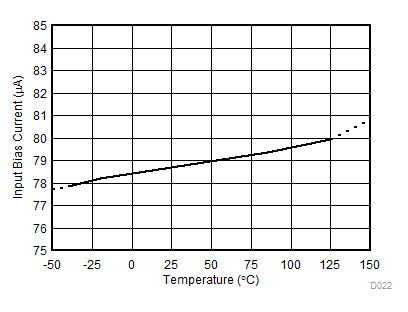Figure 22. Input Bias Current vs Temperature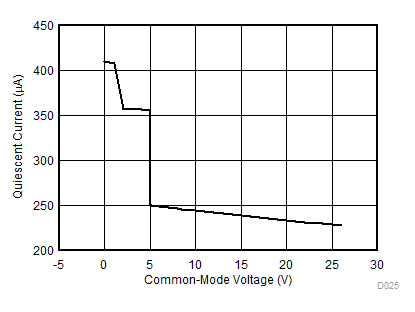Figure 24. Quiescent Current vs Common-Mode Voltage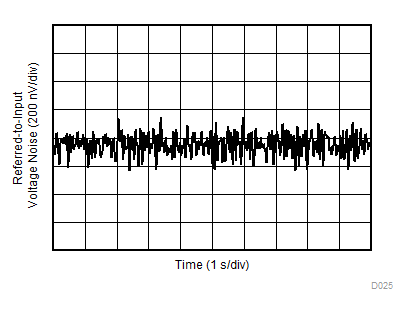Figure 26. 0.1-Hz to 10-Hz Voltage Noise (Referred-to-Input)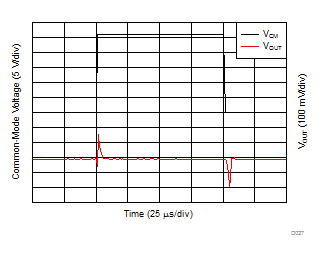Figure 28. Common-Mode Voltage Transient Response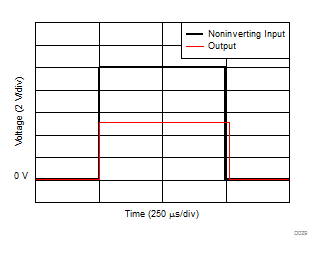Figure 30. Noninverting Differential Input Overload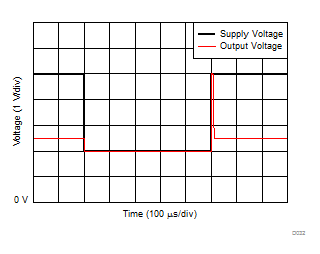Figure 32. Brownout Recovery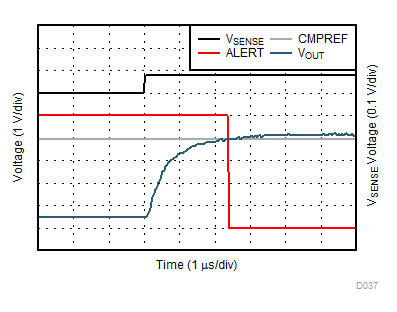Figure 34. VSENSE Voltage Response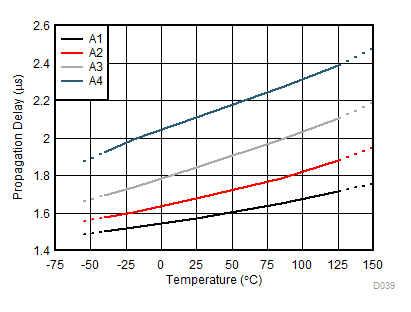Figure 36. Total Propagation Delay vs Temperature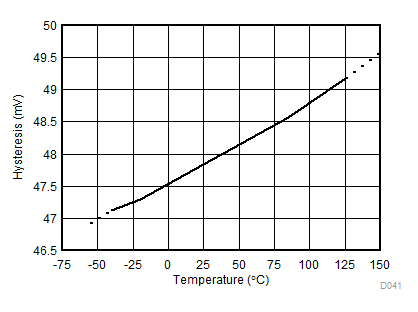Figure 38. Hysteresis vs Temperature